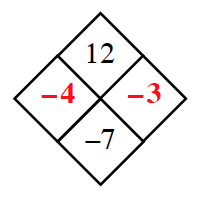### Home > INT2 > Chapter 1 > Lesson 1.1.2 > Problem1-18

1-18.

Finding and using a pattern is an important problem-solving skill necessary for your study of mathematics. The patterns in Diamond Problems are used later in the course to solve other types of algebraic problems.

Look for a pattern in the first three diamonds below. For the fourth diamond, explain how to find the missing numbers (?) if you know the two numbers (#).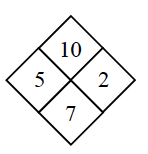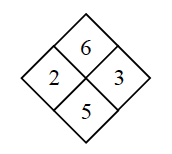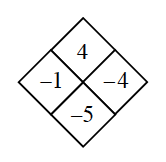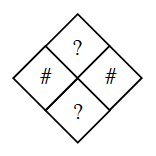Copy the Diamond Problems below onto your paper, then use the pattern you discovered to complete each one.

What do you do to each of the (#) numbers to get to the (?) numbers?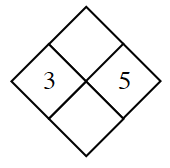Answer (a):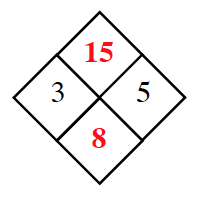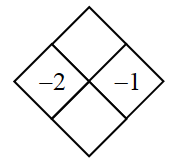Answer (b):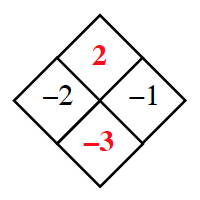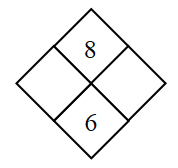Answer (c):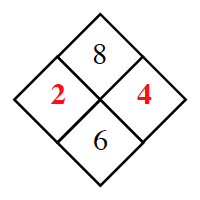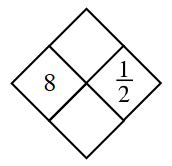Answer (d):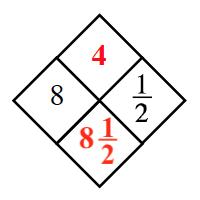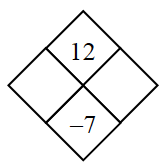Answer (e):